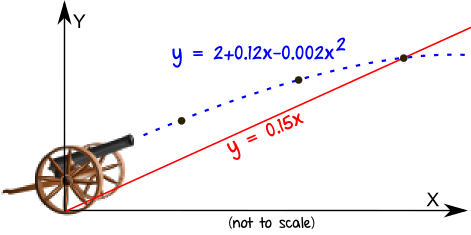New Topic: Quadratic Theory, MiA Unit 2 Ch1.2

By the end of this topic you will be able to:
• use the quadratic formula to calculate roots
• know that discriminant of ax² + bx + c = 0 is b² – 4ac
• use discriminant to determine nature of roots of a quadratic
• use discriminant to find condition that the roots of a quadratic are real, equal or unequal
• know condition for tangency; intersection of line and parabola (lines and curves)